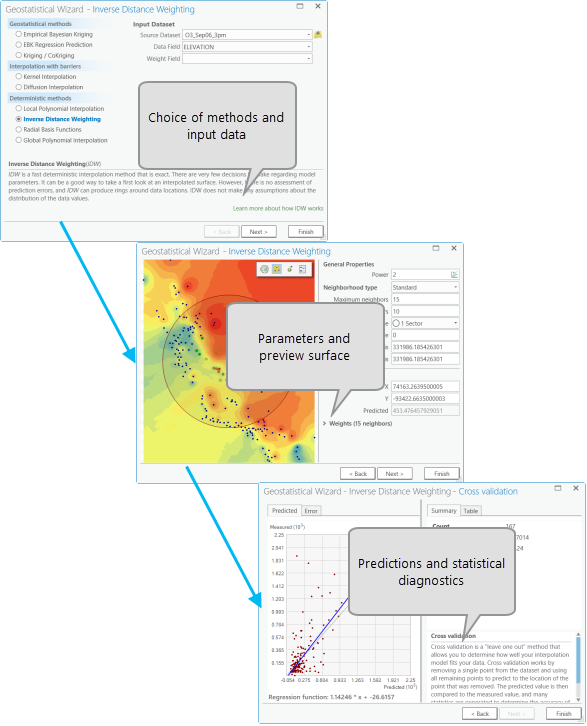# Get started with Geostatistical Analyst in ArcGIS Pro

Mit der Geostatistical Analyst-Lizenz verfügbar.

The Geostatistical Analyst extension contains a suite of tools that help you prepare your data for interpolation, fit an interpolation model, and validate the results of the interpolation. The two main components of Geostatistical Analyst are the Geostatistical Wizard and the Geostatistical Analyst toolbox.

## Geostatistical Wizard

The Geostatistical Wizard is accessed through the Analysis Ribbon tab in ArcGIS Pro.

The Geostatistical Wizard is a dynamic set of pages that is designed to guide you through the process of constructing and evaluating the performance of an interpolation model. Choices made on one page determine which options will be available on the following pages and how you interact with the data to develop a suitable model. The wizard guides you from the point when you choose an interpolation method all the way to viewing summary measures of the model's expected performance. A simple version of this workflow (for inverse distance weighted interpolation) is represented graphically below:A simple workflow in the Geostatistical Wizard

## Process of building an interpolation model

Geostatistical Analyst includes many tools for analyzing data and producing a variety of output surfaces. While the reasons for your investigations may vary, you're encouraged to adopt the approach described in Geostatistical workflow when analyzing and mapping spatial processes:

• Represent data—Create layers and display them in ArcGIS Pro.
• Explore data—Examine the statistical and spatial properties of your datasets using, for example, the histogram chart.
• Choose an appropriate interpolation method—The choice should be driven by the objectives of the study, your understanding of the phenomenon, and the available output surfaces.
• Fit the model—Perform the interpolation, possibly configuring parameters to fit the statistical properties of your data.
• Perform diagnostics—Check that the results are reasonable, and evaluate the output surface using cross-validation and validation. This helps you understand how well the model predicts the values at unsampled locations.

The Geostatistical Analyst toolbox offers many interpolation methods. You should always have a clear understanding of the objectives of your study and how the predicted values (and other associated information) help you make more informed decisions when choosing a method. To provide some guidance, see Classification trees for a set of classification trees of the diverse methods.

## Interpolation methods available in Geostatistical Analyst

The interpolation functions in Geostatistical Analyst can be divided into two categories: deterministic and geostatistical.

### Deterministic methods

Deterministic techniques have parameters that control either the extent of similarity (for example, inverse distance weighted) of the values or the degree of smoothing (for example, radial basis functions) in the surface. These techniques are not based on a random spatial process model, and there is no explicit measurement or modeling of spatial autocorrelation in the data. Deterministic methods include the following:

### Geostatistical methods

Geostatistical techniques assume at least some of the spatial variation observed in natural phenomena can be modeled by random processes with spatial autocorrelation and require that the spatial autocorrelation be explicitly modeled. Geostatistical techniques can be used to describe and model spatial patterns (variography), predict values at unmeasured locations (kriging), and assess the uncertainty associated with a predicted value at the unmeasured locations (kriging).

Learn more about kriging in Geostatistical Analyst

Empirical Bayesian kriging is available as a geoprocessing tool. The tool can be used to produce the following surfaces:

• Maps of kriging predicted values
• Maps of kriging standard errors associated with predicted values
• Maps of probability, indicating whether a predefined critical level was exceeded
• Maps of quantiles for a predetermined probability level

EBK Regression Prediction can be used to create empirical Bayesian kriging models that use explanatory variable rasters to improve the accuracy of the interpolation. GA Layer To Rasters can be used to export these models to the four output types described above.

Empirical Bayesian Kriging 3D can be used to interpolate 3D points that have an x-, y-, and z-coordinate along with a measured value to interpolate.

## Geostatistical Analyst toolbox

The Geostatistical Analyst toolbox includes tools for analyzing data, producing a variety of interpolation surfaces, examining and transforming geostatistical layers to other formats, performing geostatistical simulation and sensitivity analysis, and aiding in designing sampling networks. The tools have been grouped into five toolsets:

• Interpolation—Contains geoprocessing tools that perform interpolation used as stand-alone tools, or in ModelBuilder and Python.
• Sampling network design—Contains tools that aid in designing or modifying an existing sampling design or monitoring network.
• Simulation—Extends kriging by performing geostatistical simulation, and permits extraction of the simulated results for points or polygonal areas.
• Utilities—General-use tools to extract subsets of a dataset, perform cross-validation to assess model performance, examine sensitivity to variation in semivariogram parameters, and visually represent the neighborhoods used by the interpolation tools.
• Working with Geostatistical layers—Has tools that generate predictions for point locations, export geostatistical layers to raster and vector formats, and generate new geostatistical layers based on templates.